GeeksforGeeks App
Open AppBrowser
Continue

# How to Find Duplicate Values in Excel Using VLOOKUP?

In this article, we will look into how we can use the VLOOKUP to find duplicate values in Excel.

To do so follow the below steps:

• Let’s make two columns of different section to check VLOOKUP formula on columns: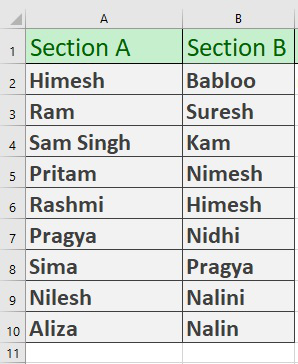Created Two Columns

• Here is the formula we are going to use:
`=VLOOKUP(List1,List2,TRUE,FALSE)`
• In this formula, the List-1 names will be searched in List-2. If there exists any duplicate name, the formula will return the name from List-1. Let`s look closely at our example for better clarification.
• In C2 we will write this formula =VLOOKUP(A2:A10,B2:B10,TRUE,FALSE) and then press enter.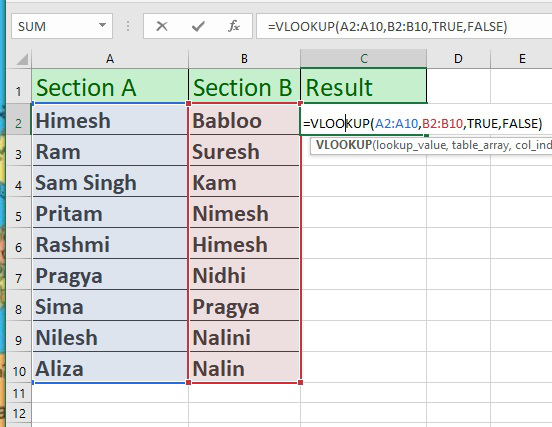• Now, we will see this result: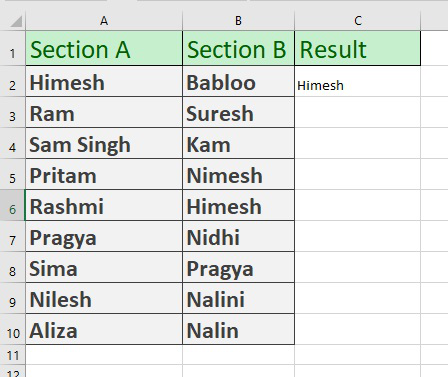Here Himesh is duplicate value

• Here the Himesh is found because the VLOOKUP function searches this name from Section A to Section B. When the same name is found it will output the result from Section A.
• Now drag the list and see all values.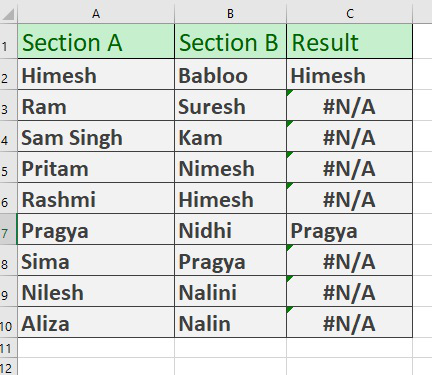Here we drag the formulated Cells

• The #N/A results are found because, in those particular cells, the names from column A are not found in column B.
• In the Result column, you’re seeing a total of 2 duplicate values ( Himesh, Pragya). #N/A values are representing the unique values of column Section A.

### Using VLOOKUP to find duplicate values in two Excel worksheets

We can also use the VLOOKUP to find duplicate values between two Excel worksheets. To do so follow the below steps:

• Make two excel workbooks Section A and Section B.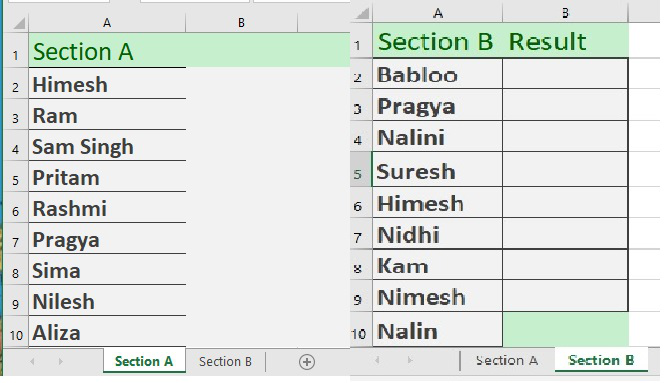Two Workbooks

• In the B2 of Section B type the below code and press enter:-
`=IF(ISERROR(VLOOKUP(A2,'Section A'!A1:A10,1,0)),"Unique","Duplicate")`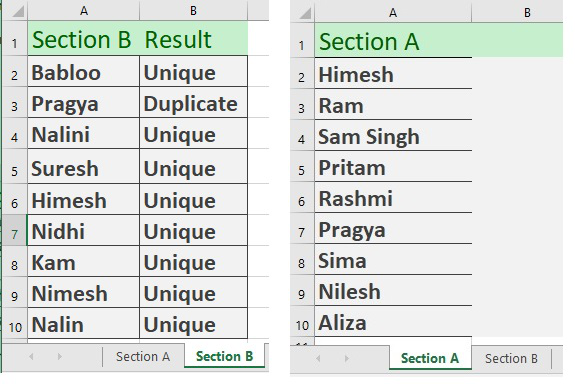Section A and Section B on different workbooks

### Using VLOOKUP to find duplicates in two Workbooks of Excel

We can also use the VLOOKUP to find duplicate values between two Excel workbooks. To do so follow the below steps:

• Now we create a new Section A in a new Workbook with different values.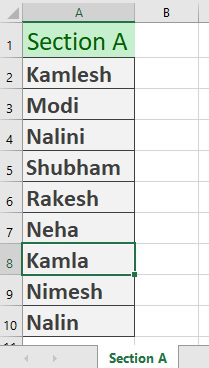New Workbook

• And In our main workbook which we were working (in our last example), create another worksheet titled Sheet 1 and again create a list of products.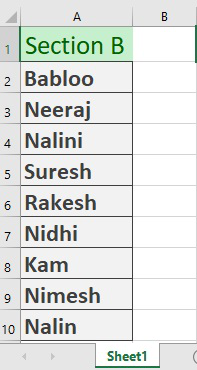Another workbook named Sheet1

• Now in cell C2 of Sheet1, write down the following formula and press enter.
`=IF(ISERROR(VLOOKUP(B2,[VL]Section A!\$A\$2:\$A\$10,1,0)),"Unique", "Duplicate")`
• You will get the result as Unique because value does not exist in Section A.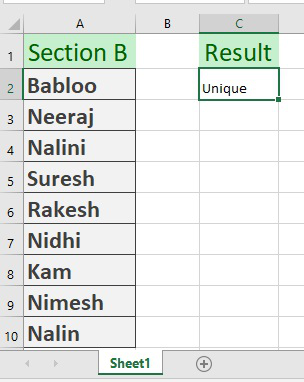Result

• Now Drag Down the formulated cell to see out the result of the whole column.
My Personal Notes arrow_drop_up